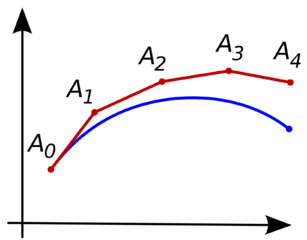Explore BrainMass
Share

# Approximation Algorithm

In Computer Science it’s often the case that finding an algorithm to solve a problem exactly will either take too long to run, or is impossible. In these cases where the problem is in NP, an approximation algorithm is used instead. Approximation algorithms are designed to find a solution that is very close to the optimal solution, but is solvable in a reasonable amount of time. While the solution found is often not the best one, with a good algorithm it can be found in polynomial time despite being NP-complete or NP-hard making it useful for real world applications.Here the red line is a computer's approximation of the unknown blue curve

An example of a well-approximated algorithm is used in finding minimum vertex covers. A vertex cover is a set of vertices on a linked graph structure that includes all the edges. When finding a minimum vertex cover, the goal is to find the least amount of vertices required. This has many real world applications in networking and topology, but since the problem is NP-Hard, computing the minimum vertex cover takes a very long time to find the exact solution. The only way to get the exact solution is by brute force, which takes exponential time meaning large graphs are essentially uncomputable. An approximation algorithm was developed for this problem that approximates the exact solution within a factor of 2, but does it in linear time. It works by selecting edges at random and putting both of its vertices into the set, and all the edges those vertices are attached to, and repeats this until all edges are in the set. The improvement in computing time means that a minimum cover can be calculated to a reasonable degree of accuracy in seconds for graphs of any size.

When crafting approximation algorithms there are several trade-offs. Often an approximation that finds a solution very quickly will be a poor approximation of the optimal solution. When designing the algorithms it is important to decided what is more important, the speed or the accuracy.

### Internet Browsers Rendering GIF and JPEG Images

Describe the GIF and JPEG file types. How are they different, and how does the size of the file relate to the speed of its presentation in the browser?

### Algorithm for Bisection method

A continuous function f(x) such that f(a) and f(b) have opposite signs, must have a zero (a point x such that f(x) = 0) in the interval [a, b]. Give a pseudocode for the bisection method algorithm Bise(f(x),a,b,error) for finding an approximation to a zero of a continuous function f(x) in the interval [a, b] accurate to withi

### Repetition

The divide-and-average algorithm for approximating the square root of any positive number a is as follows: Take any initial approximation x that is positive, and then find a new approximation by calculating the average of x and a/x, that is, (x + a/x)/2. Repeat this procedure with x replaced by this new approximation, stopping